Test: State Space Analysis

# Test: State Space Analysis

Test Description

## 10 Questions MCQ Test GATE Electrical Engineering (EE) 2023 Mock Test Series | Test: State Space Analysis

Test: State Space Analysis for Electrical Engineering (EE) 2023 is part of GATE Electrical Engineering (EE) 2023 Mock Test Series preparation. The Test: State Space Analysis questions and answers have been prepared according to the Electrical Engineering (EE) exam syllabus.The Test: State Space Analysis MCQs are made for Electrical Engineering (EE) 2023 Exam. Find important definitions, questions, notes, meanings, examples, exercises, MCQs and online tests for Test: State Space Analysis below.
Solutions of Test: State Space Analysis questions in English are available as part of our GATE Electrical Engineering (EE) 2023 Mock Test Series for Electrical Engineering (EE) & Test: State Space Analysis solutions in Hindi for GATE Electrical Engineering (EE) 2023 Mock Test Series course. Download more important topics, notes, lectures and mock test series for Electrical Engineering (EE) Exam by signing up for free. Attempt Test: State Space Analysis | 10 questions in 10 minutes | Mock test for Electrical Engineering (EE) preparation | Free important questions MCQ to study GATE Electrical Engineering (EE) 2023 Mock Test Series for Electrical Engineering (EE) Exam | Download free PDF with solutions
 1 Crore+ students have signed up on EduRev. Have you?
Test: State Space Analysis - Question 1

### Which of the following properties are associated with the state transition matrix ϕ(t)? 1. ϕ(0) = I 2. ϕ(t2 - t1) = ϕ(t2) . ϕ-1(t1) 3. ϕ(t2 + t1) = ϕ(t2) . ϕ(t1) 4. ϕ(t2 - t1) = ϕ(t2 - t0). ϕ(t0 - t1) Select the correct answer using  the codes given below:

Detailed Solution for Test: State Space Analysis - Question 1

The state transition matrix ϕ(t):
The state-transition matrix is defined as a matrix that satisfies the linear homogeneous state equation.
It represents the free response of the system.
The state-transition matrix ϕ(t) completely defines the transition of the states from the initial time t = 0 to any time t when the inputs are zero.
The state transition matrix is given by
ϕ(t) = L-1 [sI - A]-1 = eAt
Where A = state matrix
The state-transition matrix is dependent only upon the matrix A and, therefore, is sometimes referred to as the state transition matrix of A.
Properties of ϕ(t):

1. ​ϕ(0) = I
2. ϕ-1(t) = ϕ(-t)
3. ϕ(t2 - t1) = ϕ(t2 - t0). ϕ(t0 - t1)
4. ϕ(t2 + t1) = ϕ(t2) . ϕ(t1)
5. [ϕ(t)]k = ϕ(kt)
6. At t =0, dϕ / dt = A

Application:
For statement 2,
ϕ(t2 - t1) = ϕ(t2) . ϕ-1(t1)
consider
ϕ(t2 - t1) = ϕ (t2 + (-t1))
By property 4
⇒ ϕ(t2 - t1) = ϕ (t2 + (-t1)) = ϕ(t2) . ϕ(-t1)
By property 2
⇒  ϕ(t2 - t1) = ϕ(t2) . ϕ(-t1) =  ϕ(t2) . ϕ-1(t1)

Test: State Space Analysis - Question 2

### Read the statements regarding controllability and observability and mark the answer as true and false. For a system to be controllable, |Qc| = 0. For the system to be observable, |Q0| = 0. For the system to be controllable and observable, |Qc| ≠ 0 and |Qo| ≠ 0.

Detailed Solution for Test: State Space Analysis - Question 2

The correct answer is option '2'
Concept:
Controllability:
A system is said to be controllable if it is possible to transfer the system state from any initial state x(t0) to any desired state x(t) in a specified finite time interval by a control vector u(t)
Kalman’s test for controllability:
ẋ = Ax + Bu
Qc = {B AB A2B … An-1 B]
Qc = controllability matrix
If |Qc| = 0, system is not controllable
If |Qc|≠ 0, system is controllable
Observability:
A system is said to be observable if every state x(t0) can be completely identified by measurement of output y(t) over a finite time interval.
Kalman’s test for observability:
Q0 = [CT ATCT (AT)2CT …. (AT)n-1 CT]
Q0 = observability testing matrix
If |Q0| = 0, system is not observable
If |Q0| ≠ 0, system is observable.

Test: State Space Analysis - Question 3

### The transfer function G(S) = C(SI - A)-1b of the system x' = Ax + bu y = Cx + du has no pole-zero cancellation. The system

Detailed Solution for Test: State Space Analysis - Question 3

State space representation:
ẋ(t) = A(t)x(t) + B(t)u(t)
y(t) = C(t)x(t) + D(t)u(t)
y(t) is output
u(t) is input
x(t) is a state vector
A is a system matrix
This representation is continuous time-variant.
Controllability:
A system is said to be controllable if it is possible to transfer the system state from any initial state x(t0) to any desired state x(t) in a specified finite time interval by a control vector u(t)
Kalman’s test for controllability:
ẋ = Ax + Bu
Qc = {B AB A2B … An-1 B]
Qc = controllability matrix
If |Qc| = 0, system is not controllable
If |Qc| ≠ 0, the system is controllable
Observability:
A system is said to be observable if every state x(t0) can be completely identified by measurement of output y(t) over a finite time interval.
Kalman’s test for observability:
Q0 = [CT ATCT (AT)2CT …. (AT)n-1 CT]
Q0 = observability testing matrix
If |Q0| = 0, system is not observable
If |Q0| ≠ 0, system is observable.
Duality property of controllability and observability:

• If the pair (A, B) is controllable then the pair (AT, BT) is observable.
• If the pair of (A, C) is observable then the pair of (AT, CT) is controllable.

If there are no pole-zero cancellations in the transfer function then the system is completely controllable and observable.

Test: State Space Analysis - Question 4

Which of the following can be extended to time-varying systems?

Detailed Solution for Test: State Space Analysis - Question 4

The state-space analysis is a very useful technique of analyzing the control system, it is based on the concept of space and applies to the LTI system as well in non – linearly time-varying system & MIMO system.
It can be used for both continuous-time as well as discrete-time systems.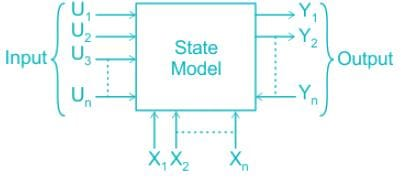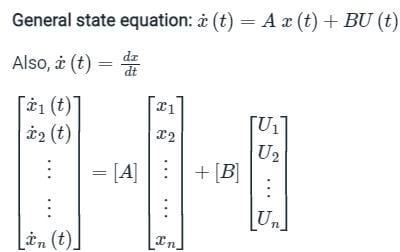Where, x1, x2, x3 …. xn are state variables
A is state matrix
B is the input matrix
Output equation: y(t) = CX(t)+DU(t)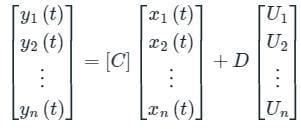Test: State Space Analysis - Question 5

Consider a system governed by the following equations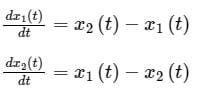The initial conditions are such that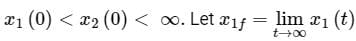and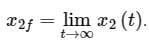Which one of the following is true?

Detailed Solution for Test: State Space Analysis - Question 5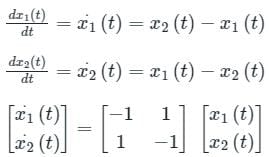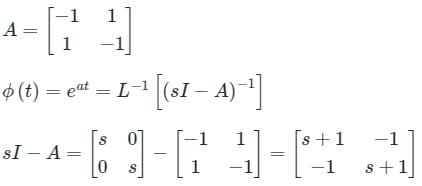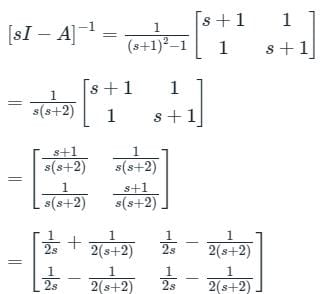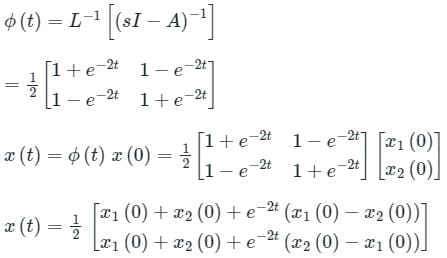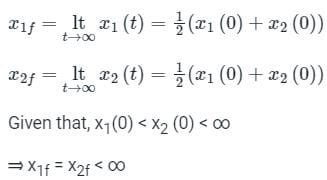Test: State Space Analysis - Question 6

State variable description of an LTI system is given by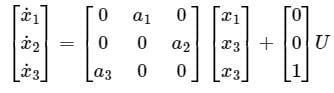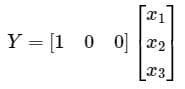Where Y is the output and u is input. System is controllable for

Detailed Solution for Test: State Space Analysis - Question 6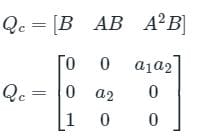System is controllable if |QC| ≠ 0
a1a2 (0 – a2) ≠ 0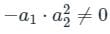Hence condition for controllability is
a1 ≠ 0, a2 ≠ 0, a3 = 0

Test: State Space Analysis - Question 7

The state transition matrix of a control system is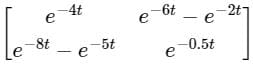. The system matrix A is

Detailed Solution for Test: State Space Analysis - Question 7

Concept:
The state transition matrix ϕ(t):
The state-transition matrix is defined as a matrix that satisfies the linear homogeneous state equation.
It represents the free response of the system.
The state-transition matrix ϕ(t) completely defines the transition of the states from the initial time t = 0 to any time t when the inputs are zero.
The state transition matrix is given by
ϕ(t) = L-1 [sI - A]-1 = eAt
Where A = state matrix
The state-transition matrix is dependent only upon the matrix A and, therefore, is sometimes referred to as the state transition matrix of A.
Properties of ϕ(t):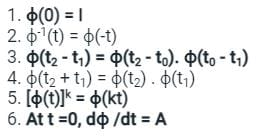Calculation:
Given that
State transition matrix ϕ(t) =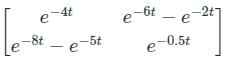Consider the sixth property of the state transition matrix
⇒ At t = 0, dϕ /dt = A
Where A is system or state matrix,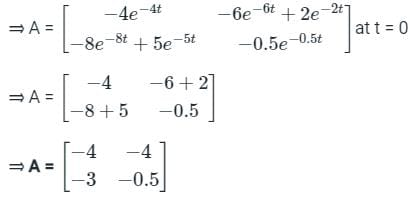Test: State Space Analysis - Question 8

Consider the following properties attributed to state model of a system:

1. State model is unique.
2. Transfer function for the system is unique.
3. State model can be derived from transfer function of the system.

Which of the above statements are correct?

Detailed Solution for Test: State Space Analysis - Question 8
• In the transfer function model, initial conditions are assumed to be zero. In the state-space model, transfer function is not the starting point for the system analysis, and it considers the initial conditions of the system.
• The transfer function for the system is unique.
• The transfer function is applicable only for linear time-invariant systems. The state-space model is applicable to linear time-variant systems also.
• State-space representation of a system is not unique, it may have more than one representation.
• State model can be derived from transfer function of the system.
Test: State Space Analysis - Question 9

The linear time invariant system is represented by the state space model as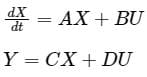Consider n=number of state variables, m = number of inputs, p= number of outputs. The state transition matrix Φ( t) Φ( t) is given by:

Detailed Solution for Test: State Space Analysis - Question 9

Concept:
The state transition matrix [ϕ(t)] is given by:
ϕ(t) = eAt = L-1 [(SI-A)]-1
where, A = System matrix
I = Identity matrix
Properties of state transition matrix:
(1) State transition matrix at t = 0 is always equal to the identity matrix.
ϕ(0) = eA0 = I
(2) The differentiation of the state transition matrix at t = 0 is always equal to its system matrix.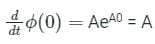Test: State Space Analysis - Question 10

A system is represented by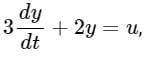what is the transfer function to the system?

Detailed Solution for Test: State Space Analysis - Question 10

Concept:
A transfer function (TF) is defined as the ratio of Laplace transform of the output to the Laplace transform of the input by assuming initial conditions are zero.
TF = L[output] / L[input]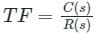For unit impulse input i.e. r(t) = δ(t)
⇒ R(s) = δ(s) = 1
Now transfer function = C(s)
Therefore, transfer function is also known as impulse response of the system.
Transfer function = L[IR]
IR = L-1 [TF]
Calculation:
Given differential equation is,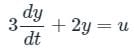Laplace transform of the above equation is given by⇒ 3s Y(s) + 2 Y(s) = U(s)
⇒ Y(s) (3s + 2) = U(s)
∴ Transfer function is given by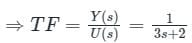## GATE Electrical Engineering (EE) 2023 Mock Test Series

22 docs|274 tests
Information about Test: State Space Analysis Page
In this test you can find the Exam questions for Test: State Space Analysis solved & explained in the simplest way possible. Besides giving Questions and answers for Test: State Space Analysis, EduRev gives you an ample number of Online tests for practice

## GATE Electrical Engineering (EE) 2023 Mock Test Series

22 docs|274 tests(Scan QR code)